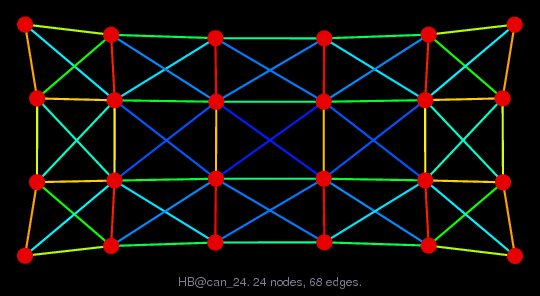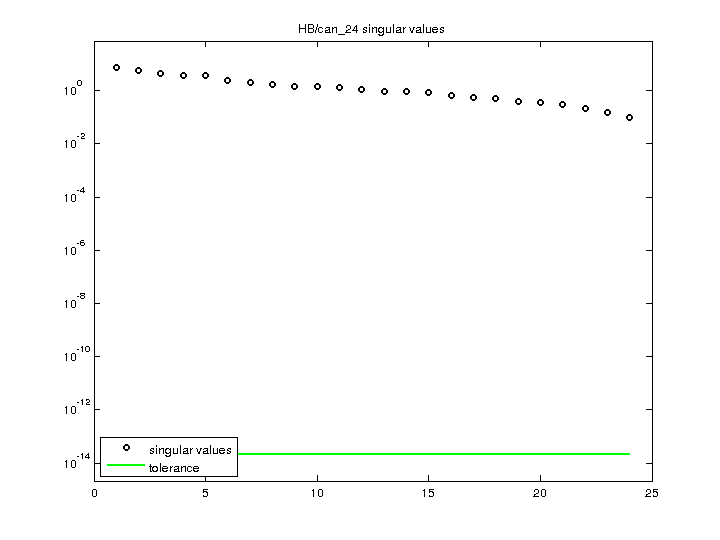Matrix: HB/can_24

Description: SYMMETRIC PATTERN FROM CANNES,LUCIEN MARRO,JUNE 1981.(undirected graph drawing)• Matrix group: HB
• download as a MATLAB mat-file, file size: 674 bytes. Use UFget(97) or UFget('HB/can_24') in MATLAB.

 Matrix properties number of rows 24 number of columns 24 nonzeros 160 structural full rank? yes structural rank 24 # of blocks from dmperm 1 # strongly connected comp. 1 explicit zero entries 0 nonzero pattern symmetry symmetric numeric value symmetry symmetric type binary structure symmetric Cholesky candidate? yes positive definite? no

 author L. Marro editor I. Duff, R. Grimes, J. Lewis date 1981 kind structural problem 2D/3D problem? yes

 Ordering statistics: result nnz(chol(P*(A+A'+s*I)*P')) with AMD 120 Cholesky flop count 6.6e+02 nnz(L+U), no partial pivoting, with AMD 216 nnz(V) for QR, upper bound nnz(L) for LU, with COLAMD 134 nnz(R) for QR, upper bound nnz(U) for LU, with COLAMD 196

 SVD-based statistics: norm(A) 7.33557 min(svd(A)) 0.0943378 cond(A) 77.7585 rank(A) 24 sprank(A)-rank(A) 0 null space dimension 0 full numerical rank? yes

 singular values (MAT file): click here SVD method used: s = svd (full (A)) status: ok